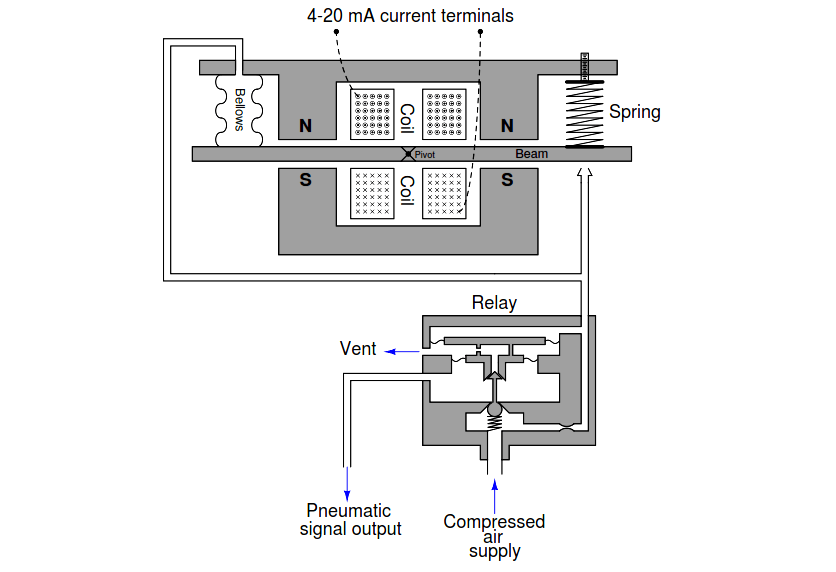# Fisher model 546 I/P transducer

Suppose this Fisher model 546 I/P transducer has an input range of 4-20 mA and an output range of 3-15 PSI:

## Fisher model 546 I/P transducerNote: This is an old model, now many smart I/P converters available n the market.

Identify which way the magnetic shunt would have to be moved in order to re-calibrate the I/P transducer to a new output range of 4-20 PSI (from 3-15 PSI), explaining your reasoning.

Identify which way the zero screw would have to be turned in order to re-calibrate the I/P transducer to a new output range of 2-14 PSI (from 3-15 PSI), explaining your reasoning.

Move the magnetic shunt further out in order to re-calibrate from 3-15 PSI to 4-20 PSI.

Turn the zero screw so the spring doesn’t push down as hard on the right-hand side of the beam in order to re-calibrate from 3-15 PSI to 2-14 PSI.

#### More Questions

1. What would happen if some of the turns in the electromagnet coil were shorted past? Would this cause a zero shift, a span shift, or a linearity shift?

2. What would happen if the zero spring broke into two separate pieces? Would this cause a zero shift, a span shift, or a linearity shift?

3. Suppose this I/P outputs a pressure of 9.0 PSI at an input current of 12.3 mA. Calculate the error, in percent of span.

4. Suppose this I/P outputs a pressure of 12.5 PSI at an input current of 16.0 mA. Calculate the error, in percent of span.

5. Suppose this I/P outputs a pressure of 5.7 PSI at an input current of 8.0 mA. Calculate the error, in percent of span.